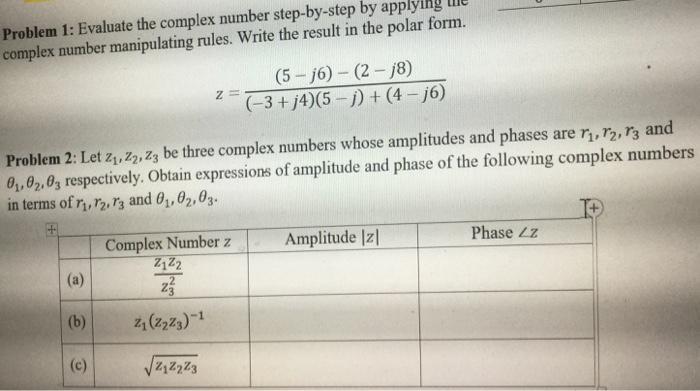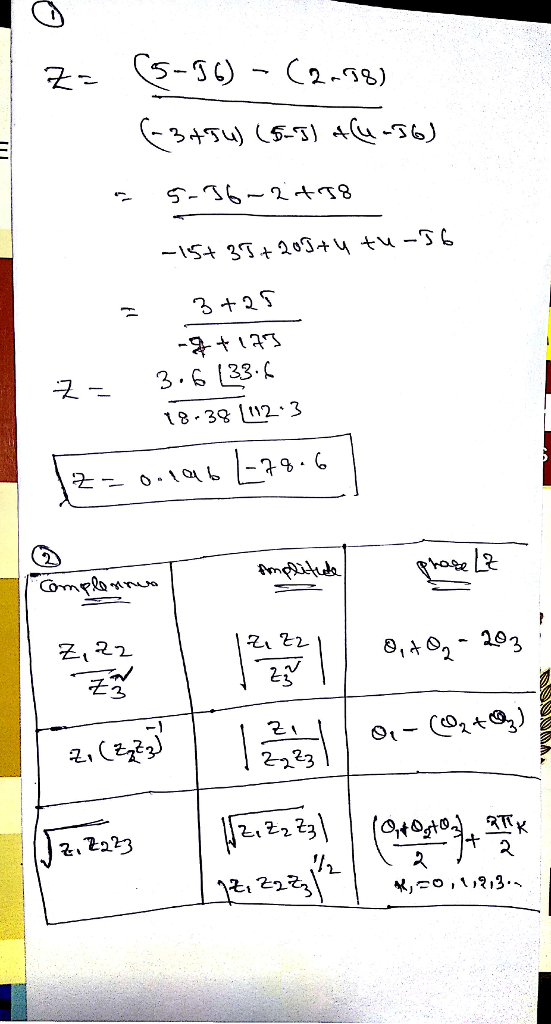# Homework Solution: Evaluate the complex number step-by-step by applying the complex number manipulating rules. Write the result in the polar form. z = (5-j6) -…Evaluate the complex number step-by-step by applying the complex number manipulating rules. Write the result in the polar form. z = (5-j6) - (2-j8)/(-3+j4)(5-j) + (4-j6) Let z_1, z_2, z_3 be three complex numbers whose amplitudes and phases are r_1, r_2, r_3 and theta_1, theta_2, theta_3 respectively. Obtain expressions of amplitude and phase of the following complex numbers in terms of r_1, r_2, r_3 and theta_1, theta_2, theta_3.